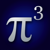# Math operation reference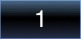#### Numbers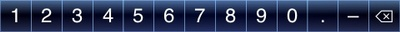When a blank operation, a number, or a variable is selected, you can start entering a number by touching the appropriate digits. The digits or decimal point that you select will be appended to the right side of the number. Touching the - menu item will switch the sign of the number from positive to negative, and vice versa.

Numbers can be edited by using the backspace menu item to delete digits, starting at the right side of the number. New digits can then be typed to replace the deleted ones.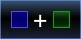#### Arithmetic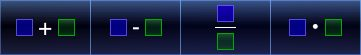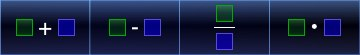Addition, subtraction, division, and multiplication are handled by this class of operations.

To add or subtract a number, tap on the relevant menu item. The highlighted side of the operation represents the currently selected item, and the outlined portion shows what will be added. For example, the far left menu item in the first set above will insert a new plus sign and blank operation to the right of the currently selected operation. Every time you tap the menu item, another plus sign and blank operation are inserted.

Two sets of items are presented for each arithmetic operation, providing for new operations to be inserted on either side of the current operation.

If you add a multiplication operation while within an addition or subtraction operation, the multiplication operation will automatically be enclosed in parentheses to make the order of calculation clear. The same will occur if an addition or subtraction operation is inserted into a multiplication.

Division operations will physically split out the numerator and denominator. Additional division operations will appear as divisions within the numerator or denominator.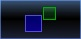#### Exponentials and logarithms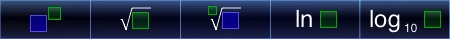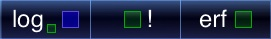This class of operations cover a range of calculation types, primarily centered around powers of numbers.

Starting from the left, the first menu item takes the selected item to an arbitrary power. For example: to square a number, select that number, tap on this item, tap on the newly created blank exponent, and type in the number 2. You can enter complex expressions in the exponent, as well, which will be evaluated into a numerical power and applied to the base operation.

The second and third menu items let you take square and arbitrary roots of your selected operation, respectively. The arbitrary root works in a similar manner to the above-described power operation, where you specify the root in the newly-created blank operation. Note that for even root powers (square, 4th root, etc.), an operation with a negative value will not evaluate to a numerical result (imaginary numbers are not yet handled by Pi Cubed).

The fourth, fifth, and sixth operations take the natural logarithm (base e), base 10 logarithm, and logarithm with an arbitrary base of the selected operation. Again, logarithms with arbitrary bases behave in the same manner as the power operation or arbitrary roots, where you can type in a numeric value for the base in the newly formed blank base operation.

The seventh menu item performs a factorial operation on the selected numerical value. This only works for operations that evaluate to an integer value.

The last menu item in this class performs the error function, a statistical operation.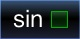#### Trigonometry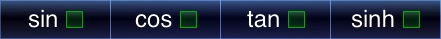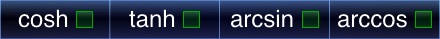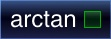This class of operations contain the standard trigonometry operations and their variants. Currently, all operations perform their calculations in degrees.

In addition to the base sine (sin), cosine (cos), and tangent (tan) operations are their hyperbolic versions (sinh, cosh, and tanh). Finally, the inverse, or arc, versions of the operations (arcsin, arccos, arctan) are included. The inverse functions take in what would normally be the result of the standard trigonometry functions and output an angle (again, currently in degrees),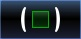#### Enclosures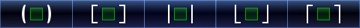Enclosures simply provide a means of visually grouping portions of a calculation. The first two variants, parentheses and brackets, have no functional differences.

The third operation in this class takes the absolute value of the contained expression.

The last two operations take the floor of an expression (the largest integer less than the expression's value) and the ceiling of an expression (the smallest integer greater than the expression's value).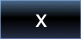#### Variables and constants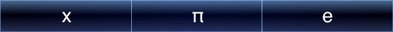Separate from the numbers that you can enter in yourself, you can also create your own variables within equations. To do this, select a blank operation and tap on the first menu item on the left. This will insert a variable with a name of "x" and a default variable description.

Additionally, two fundamental mathematical constants are provided: Pi and e. Tapping on either will replace the currently selected number with that constant.